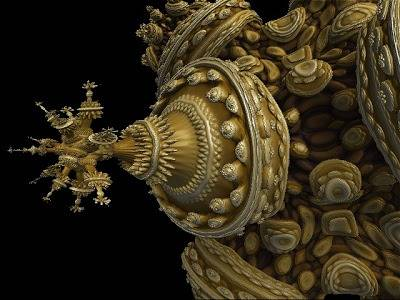# Rotation from axis to axis in 4 dimensions

• Matt Benesi
In summary, to rotate around a given vector in 3 dimensions, you need to find its rotation matrix, which is determinant 1 and has R^T = R^-1. You can do this by considering a change of basis in the matrix sense.f

#### Matt Benesi

I can easily visualize the 3 dimensional way to do so, and already have.

I'd like to rotate from the (-1,-1,-1,-1) -- (1,1,1,1) line to the x-axis (-1,0,0,0) -- (1,0,0,0).

To do so in 3 dimensions (-1,-1,-1) -- (1,1,1) line to the x-axis (-1,0,0) -- (1,0,0)

I rotated around the z axis (xy plane) by pi/2 - acos(sqrt(1/2))= pi/4

Next I rotated around the y-axis (zx plane) by pi/2 - acos (sqrt(2/3)) *** I had to use this angle because the x portion had already been rotated with the y portion

This got me to the x axis.

Should I just rotate around the xw plane?

I'm assuming the 3rd angle would be pi/2 - acos(sqrt(3/4)) because I will have to take into account the 2 previous x rotations.

The reason is I'm modifying a fractal's 4 dimensional formula:MODS- don't know if this is the correct forum?

Last edited:
Are you familiar with quaternions? The 4d analogue to quaternions is very easy to work with, and all it requires is information about the plane of rotation (which is easy to get here, since you clearly explained what you want the original and final vectors to be). There would be no need to break the rotation down into a series of rotations in unit planes.

•Matt Benesi
Hey Matt Benesi.

Basically for a rotation, you need to an axis rotate around and an angle to rotate around for.

If you look at points only you need to find a normal vector that is normal to the sub-space formed by linear combinations of the two vectors. So in point form you have (-1,-1,-1,-1) to (1, 0, 0, 0) as your start and final points, and you need a basis for this.

The basis can be found by the use of Gram-Schmidt in a modified way where you start with this two vectors and create a right-handed basis by finding for each new vector an n such that n . r = 0 where r is basically the sum of all your previous basis vectors with the rest normalized: you then choose free values for the components of n and make it satisfy that equation and then normalize that vector.

What will happen is if the orientation is kept right, you will generate a rotation matrix with determinant 1 and R^T = R^-1 that can be applied to the vector.

In terms of getting the actual transformation you will need to consider a change of basis in the matrix sense.

Basically you know that Ax = y and Bx = z where A is the basis for one system and B is for the other. But x = A^(-1)y which means BA^(-1)y = z and depending on which quantity you want, then you get y or z since both and A are invertible (and it's easy to calculate since any rotation matrix has its inverse as its tranpose).

If you keep the orientation right throughout the process, this should work for any dimension.

•Matt Benesi